# Spinor structure

(diff) ← Older revision | Latest revision (diff) | Newer revision → (diff)

on an-dimensional manifold, fibration of spin-frames

A principal fibre bundle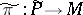overwith structure group(see Spinor group), covering some principal fibre bundle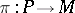of co-frames with structure group. The latter condition means that there is given a surjective homomorphism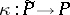of principal fibre bundles, which is the identity on the base and is compatible with the natural homomorphism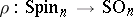. One says that the spinor structure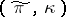is subordinate to the Riemannian metricondefined by. From the point of view of the theory of-structures, a spinor structure is a generalized-structure with structure group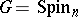together with a non-faithful representation(cf.-structure).

In a similar way one defines spinor structures subordinate to pseudo-Riemannian metrics, and spinor structures on complex manifolds subordinate to complex metrics. Necessary and sufficient conditions for the existence of a spinor structure onconsist of the orientability ofand the vanishing of the Stiefel–Whitney class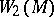. When these conditions hold, the number of non-isomorphic spinor structures onsubordinate to a given Riemannian metric coincides with the order of the group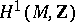(see ).

Letbe the complexification of the Clifford algebra ofwith quadratic form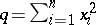. Thenhas an irreducible representation in a spaceof dimension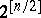, which defines a representation of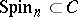in. Every spinor structureonyields an associated vector bundle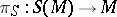with typical fibre, called a spinor bundle. The Riemannian connection ondetermines in a canonical way a connection on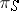. On the spaceof smooth sections of(spinor fields) there acts a linear differential operatorof order, the Dirac operator, which is locally defined by the formula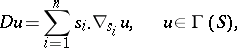where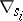() are the covariant derivatives in the directions of the system of orthonormal vector fields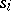and the dot denotes multiplication of spinor fields by vector fields which correspond to the above-module structure on.

Spinor fields in the kernel ofare sometimes called harmonic spinor fields. Ifis compact, then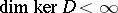, and this dimension does not change under conformal deformation of the metric . If the Riemannian metric onhas positive scalar curvature, then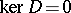(see , ).

A spinor structure on a space-time manifold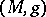(that is, on a-dimensional Lorentz manifold) is defined as a spinor structure subordinate to the Lorentz metric. The existence of a spinor structure on a non-compact space-timeis equivalent to the total parallelizability of(see ). As a module over the spinor group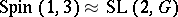, the spinor space decomposes into the direct sum of two complex-dimensional complexly-conjugate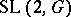-modules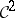and. This corresponds to the decomposition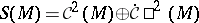of the spinor bundle, where the tensor product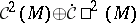is identified with the complexification of the tangent bundle. Spinor fields in space-time that are eigenfunctions of the Dirac operator characterize free fields of particles with spin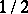, such as electrons.

How to Cite This Entry:
Spinor structure. Encyclopedia of Mathematics. URL: http://encyclopediaofmath.org/index.php?title=Spinor_structure&oldid=16824
This article was adapted from an original article by D.V. Alekseevskii (originator), which appeared in Encyclopedia of Mathematics - ISBN 1402006098. See original article